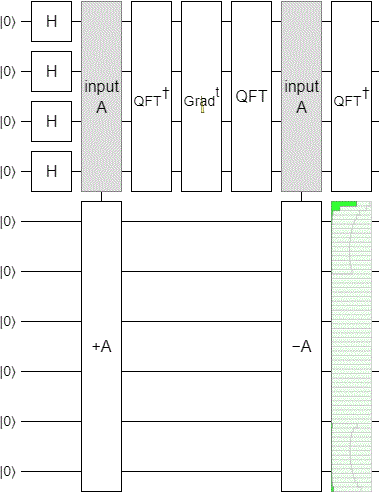# How to implement the power of a product of quantum gates as a circuit?

Suppose I have quantum gates (i.e. unitary matrices) $$A$$ and $$B$$, and I want to implement $$(AB)^x$$ in a circuit. If $$x$$ is integer, I can simply apply $$A B$$ repeatedly $$x$$-times. But what if $$x$$ is a real number? Is there a way to decompose $$(A B)^x$$ into say a product of powers of $$A$$ and $$B$$? I'm fine with some approximation.

I was thinking there might be a relation to Hamiltonian Simulation and I also looked into Trotter formulas, but could not see how to do it. In general $$A$$ and $$B$$ do not commute.

• Jun 11, 2021 at 12:09

Basically what you want is an application of phase estimation. Take a look at this paper.

To give a very crude summary. Imagine you have an eigenvector $$|\lambda\rangle$$ of a unitary $$U$$, and the eigenvalue is of the form $$e^{2\pi ip/2^t}$$. If you did phase estimation on that state, using a register of $$t$$ qubits, you'd have $$|\lambda\rangle|p\rangle$$ If you've got an arbitrary state, it'll be a superposition of terms and you can work out what'll happen by linearity. If your eigenvalue $$e^{i\phi}$$ doesn't have that exact relationship, you'll get (with high probability) the $$p$$ that is the best approximation to $$\phi 2^t/(2\pi)$$.

Now, imagine we implement a unitary on the second register $$\sum_pe^{i2\pi\theta p/2^t}|p\rangle\langle p|.$$ This is just a simply phase gate on each qubit. My output would be $$|\lambda\rangle|p\rangle e^{i2\pi\theta p/2^t}.$$ Then I can apply the inverse of phase estimation, leaving me with $$e^{i2\pi\theta p/2^t}|\lambda\rangle=U^{\theta}|\lambda\rangle.$$ You're done! Of course, you have to be careful with error terms if your eigenvalues are not exact, which is a lot of the work of the paper I linked to.

• Thanks to both of you for your nice answers. I was not aware of this application of phase estimation. I will read through your references and try to understand if this algorithm is applicable to my situation. Jun 11, 2021 at 17:07
• There's a typo in the result of applying the inverse of QPE, but its not entirely clear what the intended result was? It seems like the operator on the RHS should be $U^{\theta 2^t / 2\pi}$ for consistency with the definition of the eigenvalue of $|\lambda\rangle$ Jun 14, 2021 at 22:19

An explicit construction that you can use to apply any power of an unknown unitary $$U$$ for which you have a circuit, is to perform phase estimation of $$U$$ applied to the current state $$|\psi\rangle$$, apply a phase gradient to the phase estimation register where the strength of the phase estimation determins the power of the gate, then uncompute the phase estimation.

The main problem with this approach is that, in order to get good resolution on the phase angles, you may need to apply $$U$$ controlled by an ancilla a huge number of times.

For some operations, this is no problem at all. For example, if $$U$$ is the Fourier transform then you're golden because the Fourier transform has eigenvalues $$1, i, -1, -i$$ and each of those is a quarter turn. So you only need four applications. Also the Fourier transform squared is a simple operation, so you really only need one application to compute any fractional Fourier transform:For other operations things are more problematic. For example, suppose you apply this estimated phase phasing technique when $$U$$ is the increment operation. The $$n$$ qubit increment operation has eigenvalues of the form $$e^{k i \pi / 2^n}$$. The difference between them are of order $$2^{-n}$$, so you need $$2^n$$ controlled applications to get a good enough phase estimation. If you use fewer, you will see weird looking effects like this where the construction sorta understands how to count but... not quite:(The above circuit replaces the many controlled increments with an adder. Basically incrementing has a structure that allows you to apply high powers of it, which we call adding, and that makes the construction way cheaper than it would otherwise be. If you can apply big powers easily, you can apply tiny powers easily.)

• Thanks to both of you for your nice answers. I was not aware of this application of phase estimation. I will read through your references and try to understand if this algorithm is applicable to my situation. Jun 11, 2021 at 17:07